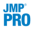Figure 2.13 Missing Value Report for Continuous Variables in Arrhythmia.jmp.
 • Missing Value Report
 • Missing Value Clustering
 • Missing Value Snapshot
 • Multivariate Normal Imputation (Not available if you entered a Numeric column with a Nominal or Ordinal modeling type in the launch window.)
 • Multivariate SVD Imputation (Not available if you entered a Numeric column with a Nominal or Ordinal modeling type in the launch window.)
 •Automated Data Imputation (Not available if you entered a Numeric column with a Nominal or Ordinal modeling type in the launch window.)
Tip: To run a missing value command across all levels of a By variable, hold down the Ctrl key and click the desired command button.
 • The dendrogram to the right of the plot shows clusters of missing data pattern rows. These are the rows that you would obtain by using Tables > Missing Data Pattern.
 • The dendrogram beneath the plot shows clusters of variables.
 • Method of imputation (either least squares or minimum-norm least squares)
 • How many values were replaced
 • Shrinkage estimator on/off
 • Factor by which the off-diagonals were scaled
 • How many rows and columns were affected
 • How many different missing value patterns there were
Click Undo to undo the imputation and replace the imputed data with missing values.
The singular value decomposition represents a matrix of observations X as X = UDV‘, where U and V are orthogonal matrices and D is a diagonal matrix.
The SVD algorithm used by default in the Multivariate SVD Imputation utility is the sparse Lanczos method, also known as the implicitly restarted Lanczos bidiagonalization method (IRLBA). See Baglama and Reichel (2005). The algorithm does the following:
 1 Each missing value is replaced with its column’s mean.
 2
 3 Each cell that had a missing value is replaced by the corresponding element of the UDV‘ matrix obtained from the SVD decomposition.
 4
 • Method of imputation
 • How many values were replaced
 • How many rows and columns were affected
Click Undo to undo the imputation and replace the imputed data with missing values.
A low-rank approximation of a matrix is of the form X = UDV‘ and can be viewed as an extension of singular value decomposition (SVD). ADI uses the Soft-Impute method as the imputation model and is designed such that the data determines the rank of the low-rank approximation.
 1 The data are partitioned into training and validation sets.
 2 Each set is centered and scaled using the observed values from the training set.
 3 For each partitioned data set, additional missing values are added within each column and are referred to as induced missing (IM) values.
 4 The imputation model is fit on the training data set along a solution path of tuning parameters. The IM values are used to determine the best value for the tuning parameter.
 5
 6